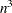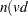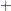Computational Resources

The CLUSTER procedure stores the data (including the COPY and ID variables) in memory or, if necessary, on disk. If eigenvalues are computed, the covariance matrix is stored in memory. If the stored distance or sorted distance algorithm is used, the distances are stored in memory or, if necessary, on disk.

With coordinate data, the increase in CPU time is roughly proportional to the number of variables. The VAR statement should list the variables in order of decreasing variance for greatest efficiency.

For both coordinate and distance data, the dominant factor determining CPU time is the number of observations. For density methods with coordinate data, the asymptotic time requirements are somewhere betweenand, depending on how the smoothing parameter increases. For other methods except EML, time is roughly proportional to. For the EML method, time is roughly proportional to.

PROC CLUSTER runs much faster if the data can be stored in memory and, when the stored distance algorithm is used, if the distance matrix can be stored in memory as well. To estimate the bytes of memory needed for the data, use the following formula and round up to the nearest multiple of d.i if density estimation or the sorted distance algorithm is used3d if stored data algorithm is used3d if density estimation is usedmax(8, length of ID variable) if ID variable is usedlength of ID variable if ID variable is usedsum of lengths of COPY variables) if COPY variables is used

where

 n is the number of observations v is the number of variables d is the size of a C variable of type double. For most computers,. i is the size of a C variable of type int. For most computers,.

The number of bytes needed for the distance matrix is.